Search IntMath
Close

450+ Math Lessons written by Math Professors and Teachers

5 Million+ Students Helped Each Year

1200+ Articles Written by Math Educators and Enthusiasts

Simplifying and Teaching Math for Over 23 Years

# Explore the slope of the tan curve

By Murray Bourne, 27 Oct 2010

UPDATE: I've created a new interactive trigonometry derivatives applet to replace the out-of-date one that was on this page.

The background below is still worth reading!

## Background

This is the 3rd article in a series. The first 2 were: Explore the slope of the sin curve and Explore the slope of the cosine curve

First, some reminders so you can better follow what is going on.

The slope of a curve means the slope of the tangent at a particular point. Now, we are going to use the word "tangent" in 2 different, but related ways.

A tangent to a curve means the line that touches the curve at one point only. (See Tangents and Normals).

A tangent is the value of the fraction opposite / adjacent in a right triangle. (See Sine, Cosine and Tangent.)

## Curve Reminders

#### Graph of tan x

Below is the curve y = tan x, in blue. Recall that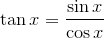Since cos x has value 0 when x = ..., -π/2, π/2, 3π/2, 5π/2, ... then our curve has discontinuities ("holes") for these x-values, since division by 0 gives an undefined result.

This means we'll have an asymptote at those values. An asymptote is a line the curve gets closer and closer to, but does not touch or intersect, shown as a red dashed line below.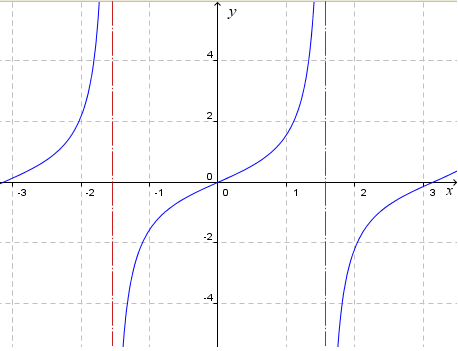y = tan x

Note: The slope of the tangent curve is positive for all values of x (except the asymptotes, of course, where the curve and the slope is undefined).

#### Graph of cos x

Next we have y = cos x. It has the same shape as the sine curve, but has been displaced (shifted) to the left by π/2 (or 90°).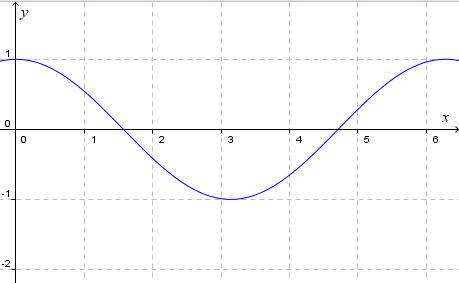y = cos x

#### Graph of sec x and sec2x

We'll also need the reciprocal of the cosine curve, which we recall is defined as the secant.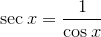To obtain the graph of y = sec x, we just take the reciprocal of each y -value of the cosine curve. So for example the point (1.05 , 0.5) (shown as a gray dot) of the cosine curve becomes (1.05, 2), (shown as a blue dot) of the secant curve.

In this graph, the cosine curve is shown in gray, and the secant curve in blue.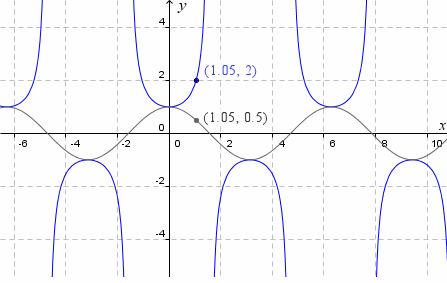y = cos x (in gray) and y = sec x (in blue)

One more step - we need the square of the secant graph values. that is, we are going to graph y = sec2x. To do that, we just take each y-value of the secant curve and square it.

So for example the point (1.05, 2) on the y = sec x curve becomes (1.05, 4) on the y = sec2x curve , as shown in the graph below.

The graph of y = sec x is shown in light blue, while the graph of y = sec2x is shown in dark blue. Of course, all the y-values of our new graph are positive, a result of squaring.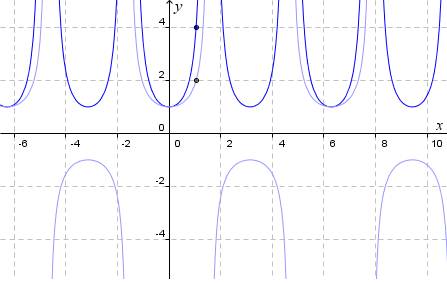y = sec x (in light blue) and y = sec2x (in dark blue)

Now we are ready to investigate tne slope of the curve y = tan x, using a GeoGebra-based JSXGraph interactive graph.

## Slope of tan x

First, have a look at the graph below and observe the slope of the (red) tangent line at the point A is the same as the y-value of the point B.

Then slowly drag the point A and observe the curve traced out by B. (The point B has the same x-value as point A, and its y-value is the same as the slope of the curve at point A).

Update: This applet has been replaced with a new one here:

Hopefully you can see that B traces out the curve y = sec2x. In other words, the slope of the graph y = tan x has value sec2x. Notice the slope is positive for all values of x.

Using calculus, we would write this result as: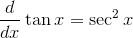See the other 2 articles in this series:

Investigate the slope of y = sin x

Investigate the slope of y = cos x

See more on the differentiation of sin, cos and tan curves. (This is in the calculus section of IntMath.)

### 2 Comments on “Explore the slope of the tan curve”

1. ly says:

it is great for me because i never known before .

2. Jenny says:

What a great resource --- and the ones for sin and cos ---- wish I had known about these before!!
THANK YOU!!!

### Comment Preview

HTML: You can use simple tags like <b>, <a href="...">, etc.

To enter math, you can can either:

1. Use simple calculator-like input in the following format (surround your math in backticks, or qq on tablet or phone):
a^2 = sqrt(b^2 + c^2)
(See more on ASCIIMath syntax); or
2. Use simple LaTeX in the following format. Surround your math with $$ and $$.
$$\int g dx = \sqrt{\frac{a}{b}}$$
(This is standard simple LaTeX.)

NOTE: You can mix both types of math entry in your comment.